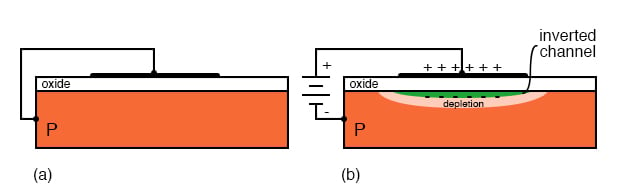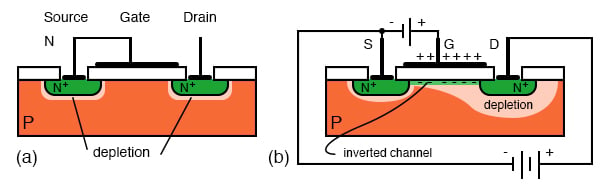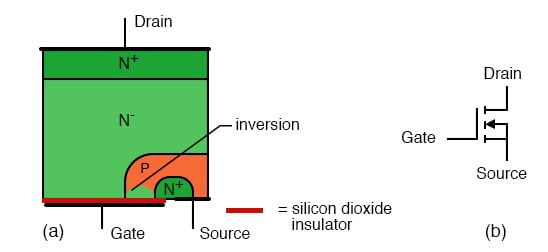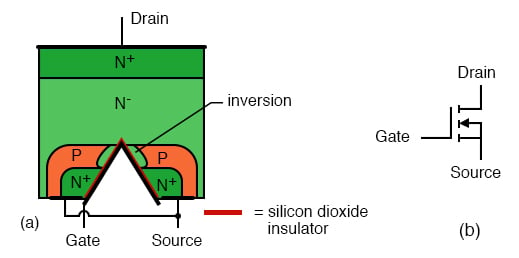# Insulated-gate Field-effect Transistors (MOSFET)

## Chapter 2 - Solid-state Device Theory

The Insulated-Gate Field-Effect Transistor (IGFET), also known as the Metal Oxide Field Effect Transistor (MOSFET), is a derivative of the field effect transistor (FET). Today, most transistors are of the MOSFET type as components of digital integrated circuits. Though discrete BJT’s are more numerous than discrete MOSFET’s. The MOSFET transistor count within an integrated circuit may approach hundreds of a million. The dimensions of individual MOSFET devices are under a micron, decreasing every 18 months. Much larger MOSFET’s are capable of switching nearly 100 amperes of current at low voltages; some handle nearly 1000 V at lower currents. These devices occupy a good fraction of a square centimeter of silicon. MOSFET’s find much wider application than JFET’s. However, MOSFET power devices are not as widely used as bipolar junction transistors at this time.

### MOSFET Operation

The MOSFET has source, gate, and drain terminals like the FET. However, the gate lead does not make a direct connection to the silicon compared with the case for the FET. The MOSFET gate is a metallic or polysilicon layer atop a silicon dioxide insulator. The gate bears a resemblance to a Metal Oxide Semiconductor (MOS) capacitor in Figure below. When charged, the plates of the capacitor take on the charge polarity of the respective battery terminals. The lower plate is P-type silicon from which electrons are repelled by the negative (-) battery terminal toward the oxide, and attracted by the positive (+) top plate. This excess of electrons near the oxide creates an inverted (excess of electrons) channel under the oxide. This channel is also accompanied by a depletion region isolating the channel from the bulk silicon substrate.N-channel MOS capacitor: (a) no charge, (b) charged.

In Figure below (a) the MOS capacitor is placed between a pair of N-type diffusions in a P-type substrate. With no charge on the capacitor, no bias on the gate, the N-type diffusions, the source and drain, remain electrically isolated.N-channel MOSFET (enhancement type): (a) 0 V gate bias, (b) positive gate bias.

A positive bias applied to the gate charges the capacitor (the gate). The gate atop the oxide takes on a positive charge from the gate bias battery. The P-type substrate below the gate takes on a negative charge. An inversion region with an excess of electrons forms below the gate oxide. This region now connects the source and drain N-type regions, forming a continuous N-region from source to drain. Thus, the MOSFET, like the FET is a unipolar device. One type of charge carrier is responsible for conduction. This example is an N-channel MOSFET. Conduction of a large current from source to drain is possible with a voltage applied between these connections. A practical circuit would have a load in series with the drain battery in Figure above (b).

### E-MOSFET

The MOSFET described above in Figure above is known as an Enhancement Mode MOSFET. The non-conducting, off, channel is turned on by enhancing the channel below the gate by application of a bias. This is the most common kind of device. The other kind of MOSFET will not be described here. See the Insulated-gate field-effect transistor chapter for the depletion mode device.

The MOSFET, like the FET, is a voltage controlled device. A voltage input to the gate controls the flow of current from source to drain. The gate does not draw a continuous current. Though, the gate draws a surge of current to charge the gate capacitance.

The cross-section of an N-channel discrete MOSFET is shown in Figure below (a). Discrete devices are usually optimized for high power switching. The N+ indicates that the source and drain are heavily N-type doped. This minimizes resistive losses in the high current path from source to drain. The N- indicates light doping. The P-region under the gate, between source and drain, can be inverted by application of a positive bias voltage. The doping profile is a cross-section, which may be laid out in a serpentine pattern on the silicon die. This greatly increases the area, and consequently, the current handling ability.N-channel MOSFET (enhancement type): (a) Cross-section, (b) schematic symbol.

The MOSFET schematic symbol in Figure above (b) shows a “floating” gate, indicating no direct connection to the silicon substrate. The broken line from source to drain indicates that this device is off, not conducting, with zero bias on the gate. A normally “off” MOSFET is an enhancement mode device. The channel must be enhanced by application of a bias to the gate for conduction. The “pointing” end of the substrate arrow corresponds to P-type material, which points toward an N-type channel, the “non-pointing” end. This is the symbol for an N-channel MOSFET. The arrow points in the opposite direction for a P-channel device (not shown). MOSFET’s are four terminal devices: source, gate, drain, and substrate. The substrate is connected to the source in discrete MOSFET’s, making the packaged part a three terminal device. MOSFET’s, that are part of an integrated circuit, have the substrate common to all devices, unless purposely isolated. This common connection may be bonded out of the die for connection to a ground or power supply bias voltage.

### V-MOSN-channel “V-MOS” transistor: (a) Cross-section, (b) schematic symbol.

The V-MOS device in (Figure above) is an improved power MOSFET with the doping profile arranged for lower on-state source to drain resistance. VMOS takes its name from the V-shaped gate region, which increases the cross-sectional area of the source-drain path. This minimizes losses and allows switching of higher levels of power. UMOS, a variation using a U-shaped groove, is more reproducible in manufacture.

REVIEW:

• MOSFET’s are unipolar conduction devices, conduction with one type of charge carrier, like a FET, but unlike a BJT.
• A MOSFET is a voltage controlled device like a FET. A gate voltage input controls the source to drain current.
• The MOSFET gate draws no continuous current, except leakage. However, a considerable initial surge of current is required to charge the gate capacitance.

RELATED WORKSHEETS: